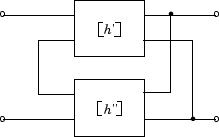# rfckt.hybrid

Hybrid connected network

## Description

Use the `hybrid` object to represent hybrid connected networks of linear RF objects characterized by the components that make up the network.

## Creation

### Syntax

``h = rfckt.hybrid``
``h = rfckt.hybrid('Ckts',value)``

### Description

````h = rfckt.hybrid` returns a hybrid connected network object whose properties all have their default values.```

example

````h = rfckt.hybrid('Ckts',value)` returns a cascaded network with elements specified in the name-value pair property `Ckts`.```

## Properties

expand all

This property is read-only.

Computed S-parameters, noise figure, OIP3, and group delay values, specified as an `rfdata.data` object. For more information, see Algorithms.

Data Types: `function_handle`

Circuit objects in network, specified as a cell array of object handles. All circuits must be 2-port. By default, this property is empty.

Data Types: `char`

This property is read-only.

Object name, specified as a `1-by-N` character array.

Data Types: `char`

This property is read-only.

Number of ports, specified as a positive integer. The default value is 2.

Data Types: `double`

## Object Functions

 `analyze` Analyze RFCKT object in frequency domain `calculate` Calculate specified parameters for rfckt objects or rfdata objects `circle` Draw circles on Smith Chart `extract` Extract specified network parameters from rfckt object or data object `listformat` List valid formats for specified circuit object parameter `listparam` List valid parameters for specified circuit object `loglog` Plot specified circuit object parameters using log-log scale `plot` Plot circuit object parameters on X-Y plane `plotyy` Plot parameters of RF circuit or RF data on X-Y plane with two Y-axes `getop` Display operating conditions `polar` Plot specified object parameters on polar coordinates `semilogx` Plot RF circuit object parameters using log scale for x-axis `semilogy` Plot RF circuit object parameters using log scale for y-axis `smith` Plot circuit object parameters on Smith chart `write` Write RF data from circuit or data object to file `getz0` Calculate characteristic impedance of RFCKT transmission line object `read` Read RF data from file to new or existing circuit or data object `restore` Restore data to original frequencies `getop` Display operating conditions `groupdelay` Group delay of S-parameter object or RF filter object or RF Toolbox circuit object

## Examples

collapse all

Create hybrid connected networks of linear RF objects with two transmission line objects using rfckt.hybrid.

```tx1 = rfckt.txline; tx2 = rfckt.txline; hyb = rfckt.hybrid('Ckts',{tx1,tx2})```
```hyb = rfckt.hybrid with properties: Ckts: {[1x1 rfckt.txline] [1x1 rfckt.txline]} nPort: 2 AnalyzedResult: [] Name: 'Hybrid Connected Network' ```

## Algorithms

The `analyze` method computes the S-parameters of the `AnalyzedResult` property using the data stored in the `Ckts` property as follows:

• The `analyze` method first calculates the h matrix of the hybrid network. It starts by converting each component network parameters to an h matrix. The following figure shows a hybrid connected network consisting of two 2-port networks, each represented by its h matrix,where

`$\begin{array}{l}\left[{h}^{\prime }\right]=\left[\begin{array}{cc}{h}_{11}{}^{\prime }& {h}_{12}{}^{\prime }\\ {h}_{21}{}^{\prime }& {h}_{22}{}^{\prime }\end{array}\right]\\ \left[{h}^{″}\right]=\left[\begin{array}{cc}{h}_{11}{}^{\prime \text{​}\prime }& {h}_{12}{}^{\prime \text{​}\prime }\\ {h}_{21}{}^{\prime \text{​}\prime }& {h}_{22}{}^{\prime \text{​}\prime }\end{array}\right]\end{array}$`

• The `analyze` method then calculates the h matrix for the hybrid network by calculating the sum of the h matrices of the individual networks. The following equation illustrates the calculations for two 2-port networks.

`$\left[h\right]=\left[\begin{array}{cc}{h}_{11}{}^{\prime }+{h}_{11}{}^{\prime \text{​}\prime }& {h}_{12}{}^{\prime }+{h}_{12}{}^{\prime \text{​}\prime }\\ {h}_{21}{}^{\prime }+{h}_{21}{}^{\prime \text{​}\prime }& {h}_{22}{}^{\prime }+{h}_{22}{}^{\prime \text{​}\prime }\end{array}\right]$`

• Finally, `analyze` converts the h matrix of the hybrid network to S-parameters at the frequencies specified in the `analyze` input argument `freq`.

 Ludwig, R. and P. Bretchko, RF Circuit Design: Theory and Applications, Prentice-Hall, 2000.